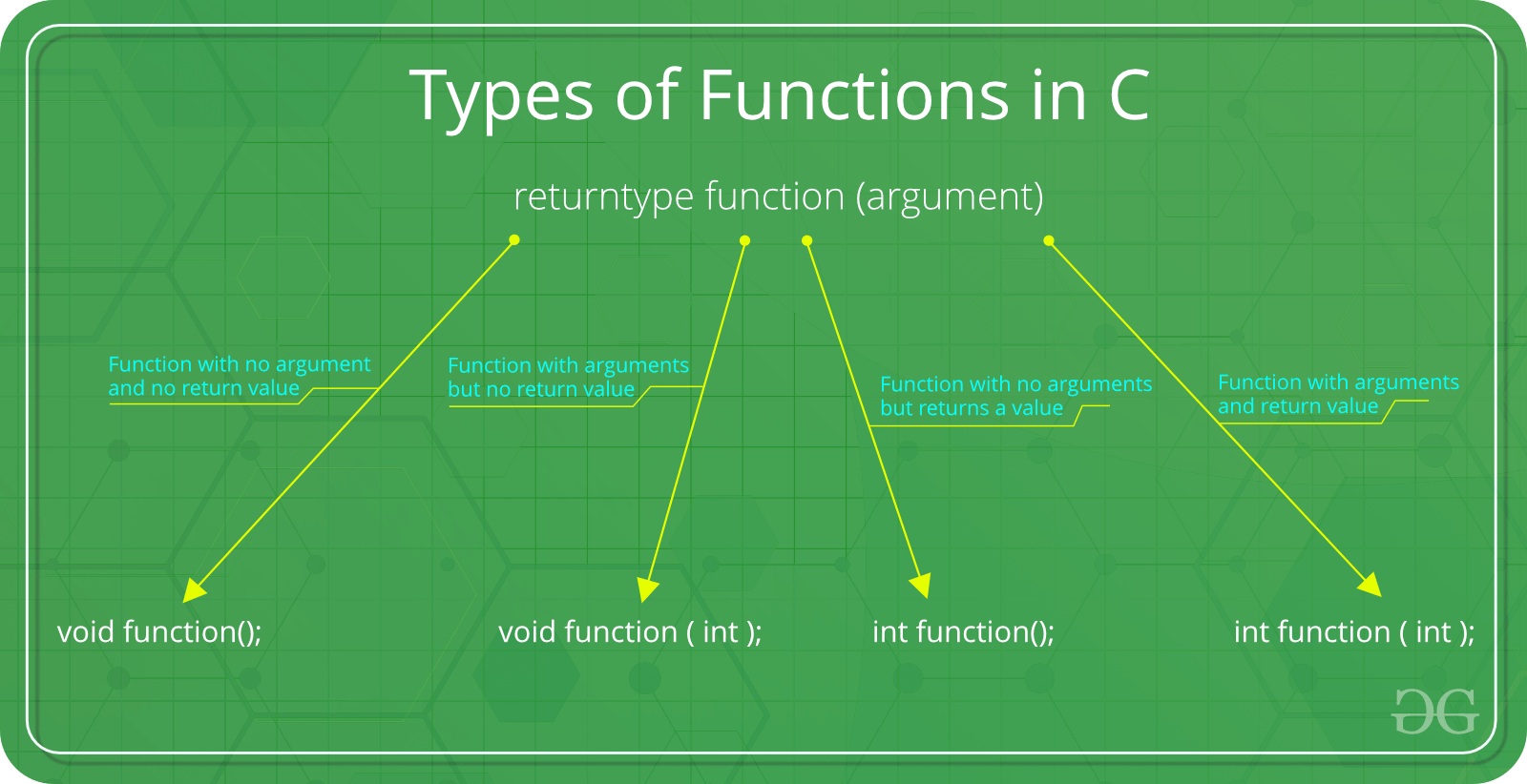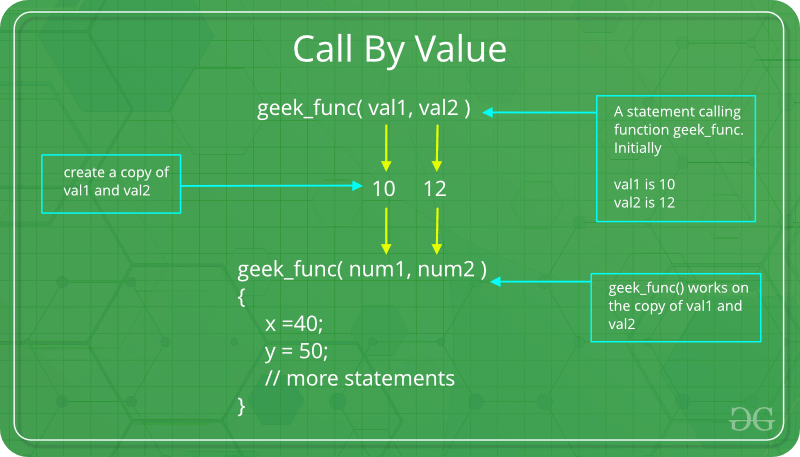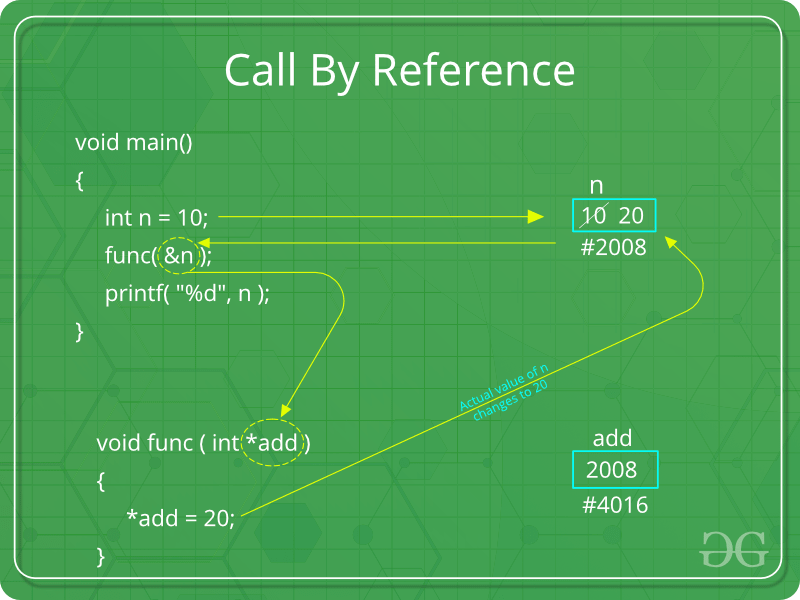Skip to content
Related Articles
AKTU 1st Year Sem 1 Solved Paper 2015-16 | COMP. SYSTEM & C PROGRAMMING | Sec B
• Last Updated : 30 Jan, 2019

Paper download link: Paper | Sem 1 | 2015-16

# B.Tech.(SEM-I) THEORY EXAMINATION 2015-16COMPUTER SYSTEM & PROGRAMMING IN C

Time: 3hrs
Total Marks: 100

Note:-

• There are three sections. Section A carries 20 marks, Section B carries 50 marks and Section C carries 30 marks.
• Attempt all questions. Marks are indicated against each question.
• Assume suitable data wherever necessary.

# Section – B

Attempt any five questions from this section: (10*5 = 50)

2. What are the different types of functions? Write a program in C to sort list of names of students in an ascending order.

• There are following categories:1. Function with no argument and no return value : When a function has no arguments, it does not receive any data from the calling function. Similarly when it does not return a value, the calling function does not receive any data from the called function.
Syntax :
```Function declaration : void function();
Function call : function();
Function definition :
void function()
{
statements;
}
```
2. Function with arguments but no return value : When a function has arguments, it receive any data from the calling function but it returns no values.

Syntax :

```Function declaration : void function ( int );
Function call : function( x );
Function definition:
void function( int x )
{
statements;
}
```
3. Function with no arguments but returns a value : There could be occasions where we may need to design functions that may not take any arguments but returns a value to the calling function. A example for this is getchar function it has no parameters but it returns an integer an integer type data that represents a character.
Syntax :
```Function declaration : int function();
Function call : function();
Function definition :
int function()
{
statements;
return x;
}
```
4. Function with arguments and return value
Syntax :
```Function declaration : int function ( int );
Function call : function( x );
Function definition:
int function( int x )
{
statements;
return x;
}
```

Program in C to sort list of names of students in an ascending order

 `#include ``#include ``#include `` ` `// Defining comparator function as per the requirement``static` `int` `myCompare(``const` `void``* a, ``const` `void``* b)``{`` ` `    ``// setting up rules for comparison``    ``return` `strcmp``(*(``const` `char``**)a, *(``const` `char``**)b);``}`` ` `// Function to sort the array``void` `sort(``const` `char``* arr[], ``int` `n)``{``    ``// calling qsort function to sort the array``    ``// with the help of Comparator``    ``qsort``(arr, n, ``sizeof``(``const` `char``*), myCompare);``}`` ` `int` `main()``{`` ` `    ``// Get the array of names to be sorted``    ``const` `char``* arr[]``        ``= { ``"geeksforgeeks"``, ``"geeksquiz"``, ``"clanguage"` `};`` ` `    ``int` `n = ``sizeof``(arr) / ``sizeof``(arr);``    ``int` `i;`` ` `    ``// Print the given names``    ``printf``(``"Given array is\n"``);``    ``for` `(i = 0; i < n; i++)``        ``printf``(``"%d: %s \n"``, i, arr[i]);`` ` `    ``// Sort the given names``    ``sort(arr, n);`` ` `    ``// Print the sorted names``    ``printf``(``"\nSorted array is\n"``);``    ``for` `(i = 0; i < n; i++)``        ``printf``(``"%d: %s \n"``, i, arr[i]);`` ` `    ``return` `0;``}`

3. Write a short note on top down program development approach.

• This approach refers to the development of program in which the program is divided into sub-programs according to tasks. These tasks are known as modules. C language supports this approach and programmers also believe that this approach is a good way to create a project in a hierarchical manner. In this the main module is developed first, then the other module and so on.

4. Write a program in C to reverse a string through pointer.

•  `#include ``#include `` ` `// Function to reverse the string``// using pointers``void` `reverseString(``char``* str)``{``    ``int` `l, i;``    ``char` `*begin_ptr, *end_ptr, ch;`` ` `    ``// Get the length of the string``    ``l = ``strlen``(str);`` ` `    ``// Set the begin_ptr and end_ptr``    ``// initially to start of string``    ``begin_ptr = str;``    ``end_ptr = str;`` ` `    ``// Move the end_ptr to the last character``    ``for` `(i = 0; i < l - 1; i++)``        ``end_ptr++;`` ` `    ``// Swap the char from start and end``    ``// index using begin_ptr and end_ptr``    ``for` `(i = 0; i < l / 2; i++) {`` ` `        ``// swap character``        ``ch = *end_ptr;``        ``*end_ptr = *begin_ptr;``        ``*begin_ptr = ch;`` ` `        ``// update pointers positions``        ``begin_ptr++;``        ``end_ptr--;``    ``}``}`` ` `// Driver code``int` `main()``{`` ` `    ``// Get the string``    ``char` `str = ``"GeeksForGeeks"``;``    ``printf``(``"Enter a string: %s\n"``, str);`` ` `    ``// Reverse the string``    ``reverseString(str);`` ` `    ``// Print the result``    ``printf``(``"Reverse of the string: %s\n"``, str);`` ` `    ``return` `0;``}`

5. Write difference between call by value and call by reference using suitable example

• There are different ways in which parameter data can be passed into and out of methods and functions. Let us assume that a function B() is called from another function A(). In this case A is called the “caller function” and B is called the “called function or callee function”. Also, the arguments which A sends to B are called actual arguments and the parameters of B are called formal arguments.

Terminology

• Formal Parameter : A variable and its type as they appear in the prototype of the function or method.
• Actual Parameter : The variable or expression corresponding to a formal parameter that appears in the function or method call in the calling environment.
• Modes:
• IN: Passes info from caller to calle.
• OUT: Callee writes values in caller.
• IN/OUT: Caller tells callee value of variable, which may be updated by callee.

Important methods of Parameter Passing

1. Pass By Value : This method uses in-mode semantics. Changes made to formal parameter do not get transmitted back to the caller. Any modifications to the formal parameter variable inside the called function or method affect only the separate storage location and will not be reflected in the actual parameter in the calling environment. This method is also called as call by value.`// C program to illustrate``// call by value``#include `` ` `void` `func(``int` `a, ``int` `b)``{``    ``a += b;``    ``printf``(``"In func, a = %d b = %d\n"``, a, b);``}``int` `main(``void``)``{``    ``int` `x = 5, y = 7;`` ` `    ``// Passing parameters``    ``func(x, y);``    ``printf``(``"In main, x = %d y = %d\n"``, x, y);``    ``return` `0;``}`

Output:

```In func, a = 12 b = 7
In main, x = 5 y = 7
```

Languages like C, C++, Java support this type of parameter passing. Java in fact is strictly call by value.
Shortcomings:

• Inefficiency in storage allocation
• For objects and arrays, the copy semantics are costly
2. Pass by reference(aliasing) : This technique uses in/out-mode semantics. Changes made to formal parameter do get transmitted back to the caller through parameter passing. Any changes to the formal parameter are reflected in the actual parameter in the calling environment as formal parameter receives a reference (or pointer) to the actual data. This method is also called as <em>call by reference. This method is efficient in both time and space.`// C program to illustrate``// call by reference``#include `` ` `void` `swapnum(``int``* i, ``int``* j)``{``    ``int` `temp = *i;``    ``*i = *j;``    ``*j = temp;``}`` ` `int` `main(``void``)``{``    ``int` `a = 10, b = 20;`` ` `    ``// passing parameters``    ``swapnum(&a, &b);`` ` `    ``printf``(``"a is %d and b is %d\n"``, a, b);``    ``return` `0;``}`

Output:

```a is 20 and b is 10
```

C and C++ both support call by value as well as call by reference whereas Java does’nt support call by reference.
Shortcomings:

• Many potential scenarios can occur
• Programs are difficult to understand sometimes

6. What are the characteristics of an algorithm? Write an algorithm to find the sum of all the numbers divisible by 3 between 11 and 50.

• Characteristics of an Algorithm
• Clear and Unambiguous: Algorithm should be clear and unambiguous. Each of its steps should be clear in all aspects and must lead to only one meaning.
• Well-Defined Inputs: If an algorithm says to take inputs, it should be well-defined inputs.
• Well-Defined Outputs: The algorithm must clearly define what output will be yielded and it should be well-defined as well.
• Finiteness: The algorithm must be finite, i.e. it should not end up in an infinite loops or similar.
• Feasible: The algorithm must be simple, generic and practical, such that it can be executed upon will the available resources. It must not contain some future technology, or anything.
• Language Independent: The Algorithm designed must be language-independent, i.e. it must be just plain instructions that can be implemented in any language, and yet the output will be same, as expected.

Algorithm to find the sum of all the numbers divisible by 3 between 11 and 50

```1. Traverse the numbers between 11 and 50
2. For each number check if it is divisible by 3
3. If divisible by 3, add them under variable sum
4. Print the sum at the end
```

7. Illustrate and compare the static and extern storage classes with a relevant example in C.

1. extern: Extern storage class simply tells us that the variable is defined elsewhere and not within the same block where it is used. Basically, the value is assigned to it in a different block and this can be overwritten/changed in a different block as well. So an extern variable is nothing but a global variable initialized with a legal value where it is declared in order to be used elsewhere. It can be accessed within any function/block. Also, a normal global variable can be made extern as well by placing the ‘extern’ keyword before its declaration/definition in any function/block. This basically signifies that we are not initializing a new variable but instead we are using/accessing the global variable only. The main purpose of using extern variables is that they can be accessed between two different files which are part of a large program. For more information on how extern variables work, have a look at this link.
2. static: This storage class is used to declare static variables which are popularly used while writing programs in C language. Static variables have a property of preserving their value even after they are out of their scope! Hence, static variables preserve the value of their last use in their scope. So we can say that they are initialized only once and exist till the termination of the program. Thus, no new memory is allocated because they are not re-declared. Their scope is local to the function to which they were defined. Global static variables can be accessed anywhere in the program. By default, they are assigned the value 0 by the compiler.
 `// A C program to demonstrate different storage``// classes``#include `` ` `// declaring the variable which is to be made extern``// an intial value can also be initialized to x``int` `x;`` ` `void` `autoStorageClass()``{`` ` `    ``printf``(``"\nDemonstrating auto class\n\n"``);`` ` `    ``// declaring an auto variable (simply``    ``// writing "int a=32;" works as well)``    ``auto` `int` `a = 32;`` ` `    ``// printing the auto variable 'a'``    ``printf``(``"Value of the variable 'a'"``           ``" declared as auto: %d\n"``,``           ``a);`` ` `    ``printf``(``"--------------------------------"``);``}`` ` `void` `registerStorageClass()``{`` ` `    ``printf``(``"\nDemonstrating register class\n\n"``);`` ` `    ``// declaring a register variable``    ``register` `char` `b = ``'G'``;`` ` `    ``// printing the register variable 'b'``    ``printf``(``"Value of the variable 'b'"``           ``" declared as register: %d\n"``,``           ``b);`` ` `    ``printf``(``"--------------------------------"``);``}`` ` `void` `externStorageClass()``{`` ` `    ``printf``(``"\nDemonstrating extern class\n\n"``);`` ` `    ``// telling the compiler that the variable``    ``// z is an extern variable and has been``    ``// defined elsewhere (above the main``    ``// function)``    ``extern` `int` `x;`` ` `    ``// printing the extern variables 'x'``    ``printf``(``"Value of the variable 'x'"``           ``" declared as extern: %d\n"``,``           ``x);`` ` `    ``// value of extern variable x modified``    ``x = 2;`` ` `    ``// printing the modified values of``    ``// extern variables 'x'``    ``printf``(``"Modified value of the variable 'x'"``           ``" declared as extern: %d\n"``,``           ``x);`` ` `    ``printf``(``"--------------------------------"``);``}`` ` `void` `staticStorageClass()``{``    ``int` `i = 0;`` ` `    ``printf``(``"\nDemonstrating static class\n\n"``);`` ` `    ``// using a static variable 'y'``    ``printf``(``"Declaring 'y' as static inside the loop.\n"``           ``"But this declaration will occur only"``           ``" once as 'y' is static.\n"``           ``"If not, then everytime the value of 'y' "``           ``"will be the declared value 5"``           ``" as in the case of variable 'p'\n"``);`` ` `    ``printf``(``"\nLoop started:\n"``);`` ` `    ``for` `(i = 1; i < 5; i++) {`` ` `        ``// Declaring the static variable 'y'``        ``static` `int` `y = 5;`` ` `        ``// Declare a non-static variable 'p'``        ``int` `p = 10;`` ` `        ``// Incrementing the value of y and p by 1``        ``y++;``        ``p++;`` ` `        ``// printing value of y at each iteration``        ``printf``(``"\nThe value of 'y', "``               ``"declared as static, in %d "``               ``"iteration is %d\n"``,``               ``i, y);`` ` `        ``// printing value of p at each iteration``        ``printf``(``"The value of non-static variable 'p', "``               ``"in %d iteration is %d\n"``,``               ``i, p);``    ``}`` ` `    ``printf``(``"\nLoop ended:\n"``);`` ` `    ``printf``(``"--------------------------------"``);``}`` ` `int` `main()``{`` ` `    ``printf``(``"A program to demonstrate"``           ``" Storage Classess in C\n\n"``);`` ` `    ``// To demonstrate auto Storage Class``    ``autoStorageClass();`` ` `    ``// To demonstrate register Storage Class``    ``registerStorageClass();`` ` `    ``// To demonstrate extern Storage Class``    ``externStorageClass();`` ` `    ``// To demonstrate static Storage Class``    ``staticStorageClass();`` ` `    ``// exiting``    ``printf``(``"\n\nStorage Classess demonstrated"``);`` ` `    ``return` `0;``}`` ` `// This code is improved by RishabhPrabhu`

8. State the features of pointers. Write a C program to sort a given number using pointers.

• Features Of Pointers:
1. Pointers save memory space.
2. Execution time with pointers is faster, because data are manipulated with the address, that is, direct access to
memory location.
3. Memory is accessed efficiently with the pointers. The pointer assigns as well as releases the memory space. Memory is dynamically allocated.
4. Pointers are used with data structures. They are useful for representing two-dimensional and multi-dimensional
arrays.
5. We can access the elements of any type of array, irrespective of its subscript range.
6. Pointers are used for file handling.
7. Pointers are used to allocate memory in a dynamic manner.
8. In C++, a pointer declared to a base class could access the object of a derived class. However, a pointer to a derived class cannot access the object of a base class. The compiler will generate an error message “cannot convert ‘A* to B*, ’” where A is the base class and B is the derived class.

9. Differentiate between the following:

• High level language and low level language:
• High Level Languages are nearly human languages. In other words, these are the languages in which the code are written in a language which are more understood by the human than by the machines.
• Low level languages are nearly machine languages. In other words, these are the languages in which the code are written in a language which are more understood by the machines than by the humans.
• Linker and Loader

Difference between Linker and Loader

LinkerLoader
Linker generates the executable module of a source program.Loader loads the executable module to the main memory for execution.
Linker takes the object code generated by an assembler, as input.Loader takes executable module generated by a linker as input.
Linker combines all the object modules of a source code to generate an executable module.Loader allocates the addresses to an executable module in main memory for execution.
The types of Linker are Linkage Editor, Dynamic linker.The types of Loader are Absolute loading, Relocatable loading and Dynamic Run-time loading.
• Logical error and Run time error
1. Run-time Errors : Errors which occur during program execution(run-time) after successful compilation are called run-time errors. One of the most common run-time error is division by zero also known as Division error. These types of error are hard to find as the compiler doesn’t point to the line at which the error occurs.
For more understanding run the example given below.
 `// C program to illustrate``// run-time error``#include ``void` `main()``{``    ``int` `n = 9, ``div` `= 0;`` ` `    ``// wrong logic``    ``// number is divided by 0,``    ``// so this program abnormally terminates``    ``div` `= n / 0;`` ` `    ``printf``(``"resut = %d"``, ``div``);``}`

Error:

```warning: division by zero [-Wdiv-by-zero]
div = n/0;```

In the given example, there is Division by zero error. This is an example of run-time error i.e errors occurring while running the program.

2. Logical Errors : On compilation and execution of a program, desired output is not obtained when certain input values are given. These types of errors which provide incorrect output but appears to be error free are called logical errors. These are one of the most common errors done by beginners of programming.
These errors solely depend on the logical thinking of the programmer and are easy to detect if we follow the line of execution and determine why the program takes that path of execution.
 `// C program to illustrate``// logical error``int` `main()``{``    ``int` `i = 0;`` ` `    ``// logical error : a semicolon after loop``    ``for` `(i = 0; i < 3; i++)``        ``;``    ``{``        ``printf``(``"loop "``);``        ``continue``;``    ``}``    ``getchar``();``    ``return` `0;``}`

No output

My Personal Notes arrow_drop_up